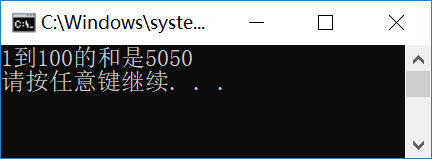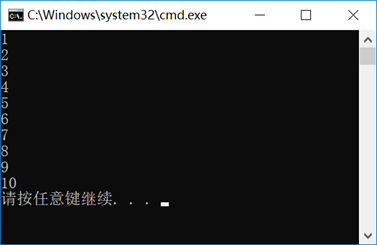``````static void Main(string[] args)
{
int sum = 0;
for( int i=1;i<=100;i++)
{
sum = sum + i;
}
Console.WriteLine("1到100的和是{0}", sum);
}
````````````for(<循环变量初始化> ; <循环条件> ; <循环变量每次变化>)
{
<语句块>
}
``````

for语句结构的含义是，循环控制变量初始化为一定的值，当其满足一定的条件时就执行<语句块>的内容，每次执行后需要一个改变循环变量数据的操作。

foreach语句结构可以方便的访问集合（数组、列表等类型，后续会讨论相关内容）中的成员，如下面的代码，我们分别使用for语句结构和foreach语句结构访问一个数组的成员。

``````static void Main(string[] args)
{
int[] arr = new int;
// 给数组成员赋值
for( int i=0;i<10;i++)
{
arr[i] = i + 1;
}
// 显示数组成员数据
foreach(int num in arr)
{
Console.WriteLine(num);
}
}
````````````while(<条件表达式>)
{
<语句块>
}
``````

``````do
{
<语句块>
}while(<条件表达式>);
``````

``````static void Main(string[] args)
{
int sum = 0, n = 1;
while (n <= 100)
{
sum += n;
n++;
}
Console.WriteLine("1到100累加结果为{0}", sum);
}
``````

``````static void Main(string[] args)
{
int sum = 0, n = 1;
do
{
sum += n;
n++;
} while (n <= 100);
Console.WriteLine("1到100累加结果为{0}", sum);
}
``````

``````static void Main(string[] args)
{
int sum = 0;
for(int i=2;i<=100;i+=2)
{
sum += i;
}
Console.WriteLine("1到100中偶数的和是{0}", sum);
}
``````

``````static void Main(string[] args)
{
int sum = 0;
for(int i=2;i<=100;i++)
{
if (i % 2 == 1) continue;
sum += i;
}
Console.WriteLine("1到100中偶数的和是{0}", sum);
}
``````

``````static void Main(string[] args)
{
int x=17; // 大于1的整数
int max = (int)Math.Sqrt(x);
bool isPrime = true;
for(int i=2;i<=max;i++)
{
if(x % i ==0 )
{
isPrime = false;
break;
}
}
Console.WriteLine("{0}{1}质数", x,
isPrime?"是":"不是");
}
``````

``````static void Main(string[] args)
{
int counter = 0;
for (int i = 0; i <= 100; i++)
{
for (int j = 0; j <= 100; j++)
{
for (int k = 0; k <= 100; k++)
{
counter++;
if (i == 10 && j == 20 && k == 30)
goto I_FOR_END;

}
}
}
I_FOR_END:;
Console.WriteLine("内层循环执行{0}次", counter);
}
``````

CHY软件小屋原创作品！